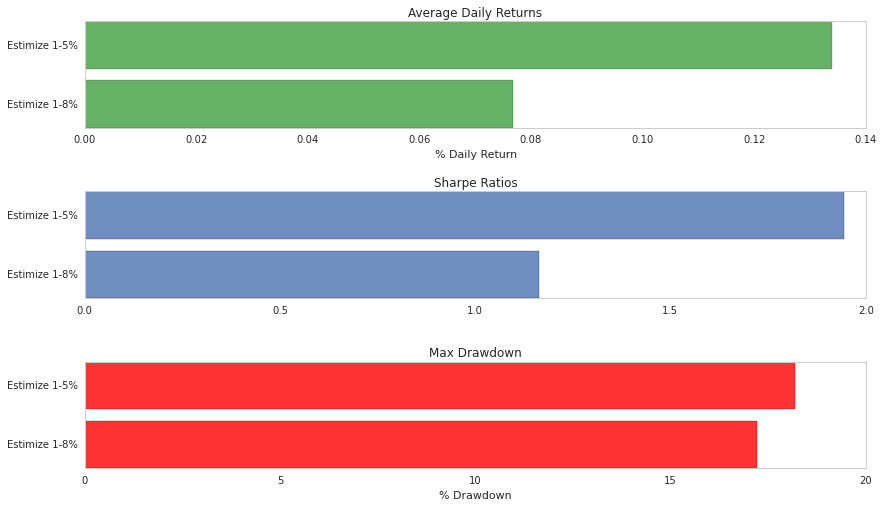Notebook

# Estimize in Quantopian: Improving your Algos with Earnings Predictions¶

Earnings estimates (earnings per share or EPS) and revenue estimates are heavily used in both quant and fundamental stock analysis as forward-looking indicators of stock performance and sources of alpha. Traditionally, estimates are given by sell-side analysts on Wall Street and are then aggregated and averaged into what's commonly referred to as "the Wall Street Consensus" or simple "the street's" expectations. Starting in 2011 however, the fintech startup Estimize launched a new platform allowing anyone on the web to share their own earnings and revenue estimates. Website visitors and contributors can browse the estimates submitted by other users.

So in collaboration with Estimize, Quantopian took this crowdsourced earnings data and created both an algorithm and a data analysis notebook to help you understand how the two development environments go hand-in-hand.

That being said, this notebook is going to cover a multitude of concepts:

By the end of this notebook you'll have

• Understood how to recreate a whitepaper in Research
• Analyze the results of multiple backtests and compare them against each other

# Finance Basics: EPS, Wall Street Consensus, and Earnings Surprise¶

• EPS (Earnings Per Share): (Net_Income - Dividends)/Shares Oustanding
• Wall Street Consensus: Aggregated consensus of all analysts in Wall Street (mostly sell-side)
• Earnings Surprise: When earnings announcements do better than, or worse than, the Wall Street Consensus

So to give you a bit of context, I'm going to show you what an earnings surprise actually looks like. This is Apple's Q2 Earnings for 2014. You'll notice that the Street's consensus was 1.46 but Apple's actual earnings landed at 1.66. That's a surprise of over 13% and as a byproduct of that, it looks like the stock price of Apple shoots up!# Recreating a whitepaper¶

Estimize makes a few claims regarding the accuracy of their data in a whitepaper they released back in September 24, 2013 and I'm going to try and replicate that:

• Claim #1: More accurate "65% of the time when there are 20 or more contributors to the Estimize Consensus."
• Claim #2: Average absolute error of Estimize Consensus is smaller than the Wall Street Consensus by 12 basis points when contributors are greater than 20

So what I'm going to do in this notebook is actually take you step-by-step and show you how to recreate a whitepaper (like the one above) within the context of our Research platform.

# Looking at Claim #1: Accuracy¶

In this case, accuracy is determined by whether or not Estimize's contributors correctly guessed the direction of the earnings surprise. So a few simple heuristics to gauge that are as follows:

• Did Estimize numbers land higher than the Wall Street Consensus when it was a positive surprise?
• Did Estimize numbers land lower than the Wall Street Consensus when it was a negative surprise?In the example above, Estimize correctly guessed the direction of the surprise as it's earnings estimates landed closer towards the actual earnings as compared with the Street's consensus.

# First Step: Wrangling the Data and Getting a Bird's Eye View¶

In :
#: Import any necessary packages
import numpy as np
import pandas as pd
import matplotlib.pyplot as pyplot

plt = pyplot

def fix_df(df):
df = df.rename(columns={'eps.actual': 'actual_eps',
'revenue.actual': 'actual_rev',
'eps.wallstreet': 'wallstreet_eps',
'revenue.wallstreet': 'wallstreet_rev',
'estimize_eps_consensus': 'estimize_eps',
'estimize_revenue_consensus': 'estimize_rev',
'number_of_estimates': 'num_participants'})
df = df[np.isfinite(df['estimize_eps'])]
return df

accuracy_dataframe = local_csv('estimize_data.csv')
accuracy_dataframe = fix_df(accuracy_dataframe)

#: Peaking at the first couple rows of our DataFrame

                          id             instrument_id  \
12  4e671c877cb02d7af700002d  4e3a3fca7aaa020432000873
13  4e671c877cb02d7af700002e  4e3a3fca7aaa020432000873
14  4e671c877cb02d7af700002f  4e3a3fca7aaa020432000873
15  4e671c877cb02d7af7000030  4e3a3fca7aaa020432000873
25  4e671c887cb02d7af700005b  4e3a3fd47aaa02043200146a

sector                      industry ticker  fiscal_year  \
12  Information Technology                      Software    SWI         2012
13  Information Technology                      Software    SWI         2012
14  Information Technology                      Software    SWI         2012
15  Information Technology                      Software    SWI         2012
25  Information Technology  Internet Software & Services    ENV         2012

fiscal_quarter  actual_eps  actual_rev  wallstreet_eps  wallstreet_rev  \
12               1        0.30       59.67           0.260          56.358
13               2        0.33       64.04           0.270          59.986
14               3        0.37       71.72           0.310          68.000
15               4        0.36       73.50           0.326          70.712
25               4        0.10       44.60           0.100          43.906

estimize_eps  estimize_rev  num_participants                 reports_at
12      0.278750     56.905000                16  2012-04-26T06:00:00-04:00
13      0.294000     61.199000                10  2012-07-25T06:00:00-04:00
14      0.338889     69.255556                 9  2012-10-25T06:00:00-04:00
15      0.355833     71.961667                12  2013-02-04T16:00:00-05:00
25      0.100000     43.992000                 5  2013-02-14T16:00:00-05:00

In :
#: Now we're going to define a Python function that takes in a Dataframe row.
def correct_prediction(row):

#: Extracting the variables beforehand to save us some typing later on
estimize = row['estimize_eps']
wallstreet = row['wallstreet_eps']
actual = row['actual_eps']

#: Defining the logic for our new column
if wallstreet < estimize and estimize < actual:
return True
elif actual < estimize and estimize < wallstreet:
return True
elif estimize < actual and actual < wallstreet and abs(estimize - actual) < abs(wallstreet - actual):
return True
elif wallstreet < actual and actual < estimize and abs(estimize - actual) < abs(wallstreet - actual):
return True
else:
return False

In :
#: We're going to use Dataframe.apply(lambda row: correct_prediction(row))
accuracy_dataframe['correct_prediction'] = accuracy_dataframe.apply(lambda row: correct_prediction(row), axis=1)

#: Use a histogram to graph the results quickly against each other
accuracy_dataframe['correct_prediction'].hist(bins=3, alpha=.6, color='#348ABD', figsize=(14,10))

#: Pretty up our graphs
plt.xticks([.2, .8], ["Incorrect", "Correct"], fontsize=20)
plt.xlabel("Type of result", fontsize=20)
plt.ylabel("Number of occurences", fontsize=20)
plt.title("The number of times Estimize correctly predicted the direction of the surprise", fontsize=20)

Out:
<matplotlib.text.Text at 0x7ff9f24d2a90>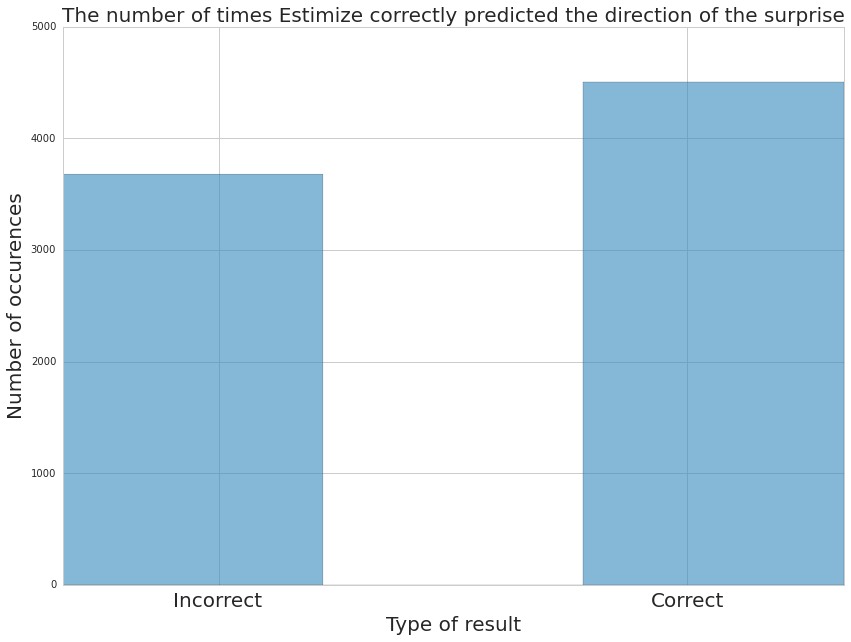So it looks like on the whole, Estimize's consensus numbers correctly predicted the direction of the surprise more often than it was wrong. But really, this is almost like 50/50. What you really want to look at is how the number of participants (the number of people giving their estimates to Estimize for a given earnings announcement) affects the accuracy of the data. This means that if having more participants translates into a more accurate estimate, I can use that as a proxy to filter down the data before testing its validity as a trading signal.

Now, in order to start looking at the possible relationship between num_participants and accuracy, the data needs to be filtered down into something that contains the average accuracy rate per participant number. So by that I mean, I need to have an average accuracy rate for all estimates with 1 participant, 2 participants, 3 participants, and etc. Pandas makes this very easy through something called groupby.

In :
"""
This cell contains the two graphing functions:
- plot_graph : Graphs the accuracy rate against the number of participants
- pretty_plot : Graphs the bar chart showing two bins (N < 19) and (N > 20)

This cell only contains the functions, they are executed later on
"""

#: Graphs the accuracy rate against the number of participants
def plot_graph(x_axis, y_axis):

#: Using the dataframe that we first loaded in, we're going to use Panda's groupby method
num_participants = accuracy_dataframe.groupby('num_participants')

for num_participant, group in num_participants:

#: Removing areas where the sample size for each participant is less than 7
if group['estimize_eps'].count() < 7:
continue

#: Getting the total number and finding a percentage
total_accuracy = group['correct_prediction'].value_counts()
percentage_more_accurate = total_accuracy[True]/(total_accuracy.sum() + 0.0)

#: Adding percentage and number of participants to y-axis and x-axis
x_axis.append(num_participant)
y_axis.append(percentage_more_accurate)

plt.figure(figsize=(12,12))

#: Plot the 65% accuracy line
plt.axhline(y=.65,color='k', ls='dashed')

#: Plotting the raw results
plt.scatter(x_axis, y_axis, alpha=0.6, color='#348ABD', lw=3, label='RAW')

#: Plotting a linear regression to fit our results
m, b = np.polyfit(x_axis, y_axis, 1)
plt.plot(x_axis, m*np.array(x_axis) + b, alpha=0.6, color='#A60628', lw=3, label='Linear Reg')

#: Pretty makeovers
plt.ylabel("% of time Estimize predicted surprise", fontsize=20)
plt.xlabel("Number of Estimize participants", fontsize=20)
plt.title("Estimize accuracy versus Wall Street Consensus", fontsize=20)
plt.legend(loc='best')
return x_axis, y_axis

#: Plots the bar chart we have in the second chart
def pretty_plot(e_avg, e_avg_20):
plt.xlabel("Number of Participants", fontsize=20)
plt.ylabel("Percentage more accurate", fontsize=20)
plt.ylim([0, 1])
plt.xlim([0, 1.6])
plt.yticks([e_avg, e_avg_20, .50, .8])
plt.axhline(y=e_avg_20,color='k', ls='dashed')
plt.axhline(y=e_avg, color='k', ls='dashed')
plt.title("Estimize Consensus Accuracy compared to Wall Street Consensus", fontsize=20)
plt.xticks([.3, 1.3], ["N < 19", "N > 20"], fontsize=20)

In :
"""
- plot_graph finds the average number of correct/incorrect predictions per num_participant
- assigns num_participants to the x_axis and the corresponding true/false rate to the y_axis
"""
x_axis = []
y_axis = []

#: Use the plot_graph function to plot our accuracy rate against number of participants
x_axis, y_axis = plot_graph(x_axis, y_axis)

#: Find the average accuracy rate according to N < 19 and N >= 20
results = dict([(x_axis[i], y_axis[i]) for i, y in enumerate(x_axis)])
one_nineteen = []
twenty_up = []
for i, v in results.iteritems():
if i < 20:
one_nineteen.append(v)
else:
twenty_up.append(v)
e_avg = (np.mean(one_nineteen)) # N < 19
e_avg_20 = (np.mean(twenty_up)) # N >= 20

#: Plot a bar chart with our new averages
plt = pyplot
N = 2
nums = (e_avg, e_avg_20)

ind = (0, 1)  # the x locations for the groups
width = 0.6     # the width of the bars

#: Take that knowledge and plot a bar chart
plt.figure(figsize=(16,12))
plot = plt.bar(ind, nums, width, color='r', alpha=.5)

#: Use the pretty plot function to graph the bar chart
pretty_plot(e_avg, e_avg_20)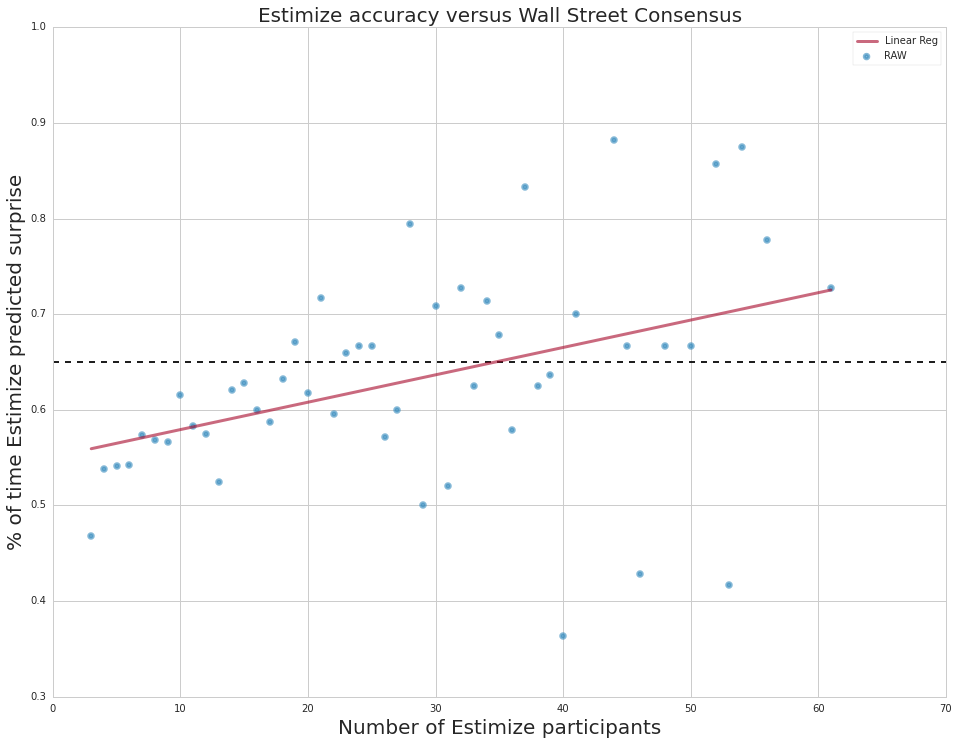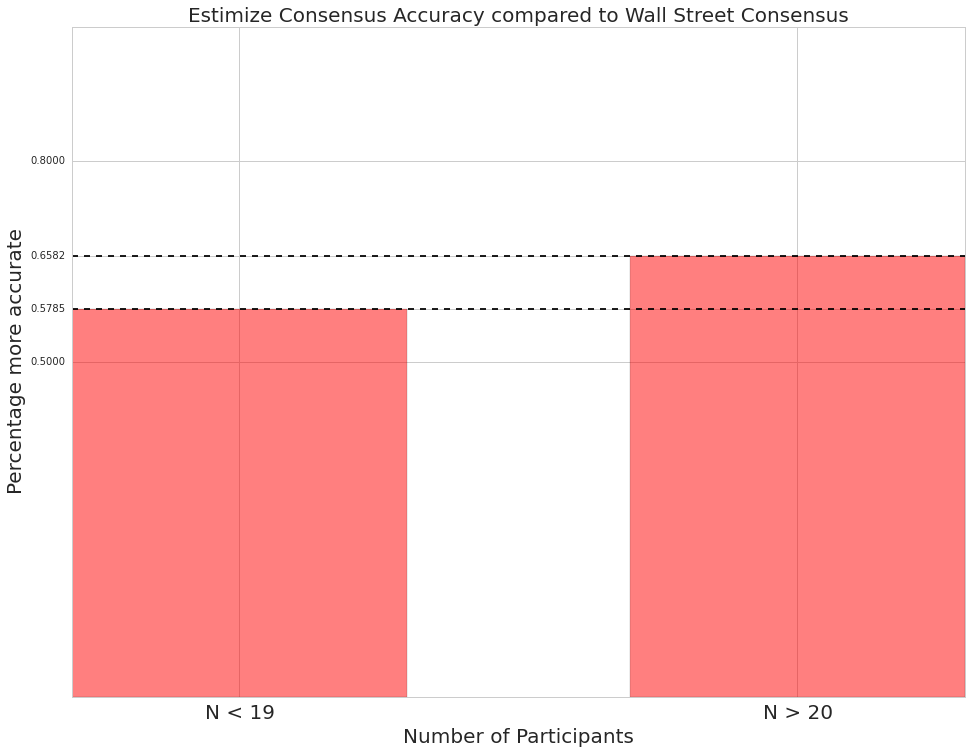So now I'm getting somewhere. The first graph shows some correlation between the number of participants and the general accuracy level of a single Estimize estimate. Just to restate, accuracy, in this case, is defined by whether or not the crowdsourced consensus numbers correctly determined the direction of the earnings surprise. To be more specific, the second graph (the red bar chart) shows that as the number of participants increases past 19, the Estimize consensus numbers are, on average, 65% more accurate than the Street in predicting the direction of an Earnings Surprise.

# Looking at Claim #2: Error Rates¶

"Average absolute error of Estimize Consensus is smaller than the Wall Street Consensus by 12 basis points when contributors are greater than 20"

• Instead of absolute error, I'm going to take you through finding the percentage error to put this all in a relative scale

Just like before, I'm going to get my dataset into a state where I've grouped by the number of participants. However, instead of using accuracy, I'm now going to look at the actual error (just how divergent are the Street's and Estimize's numbers from the actual announcement?).

In :
"""
"""
a_df = local_csv('estimize_data.csv')
a_df = fix_df(a_df)

#: Create a new column with the relative perctange error
a_df['estimize_delta'] = abs(a_df['actual_eps'] - a_df['estimize_eps'])/a_df['actual_eps']
a_df['wallstreet_delta'] = abs(a_df['actual_eps'] - a_df['wallstreet_eps'])/a_df['actual_eps']

In :
"""
Function that takes in the x_axis, y_axis, and wallstreet_y lists (empty lists)
and populates them with the average percentage error for each given number of participants
"""

def get_axes(x_axis, y_axis, wallstreet_y):
num_participants = a_df.groupby('num_participants')

#: For each num_participant iterate over them
for num_participant, group in num_participants:

#: Skip any Series (group is a Series) with a sample size of less than 7
if group['estimize_delta'].count() < 7:
continue

#: Marking the number of times that Estimize is more accurate (smaller delta) than Wall Street
estimize_delta = group['estimize_delta'].dropna()
wallstreet_delta = group['wallstreet_delta'].dropna()
avg_estimize = np.average(estimize_delta)
avg_wallstreet = np.average(wallstreet_delta)

#: Make sure that we have a valid average, if not, skip
if np.isfinite(avg_estimize) != True or np.isfinite(avg_wallstreet) != True:
continue

#: Adding percentage and number of participants to y-axis and x-axis
x_axis.append(num_participant)
y_axis.append(avg_estimize)
wallstreet_y.append(avg_wallstreet)
return x_axis, y_axis, wallstreet_y

In :
"""
The functions defined in this cell simply get the average percentage error according to the
number of participants ( N < 19 and N >= 20 ). This is very similar to how we did it in the first claim
"""

def get_averages(x_axis, y_axis):
#: Find the averages for the y_axis data
results = dict([(x_axis[i], y_axis[i]) for i, y in enumerate(x_axis)])
one_nineteen = []
twenty_up = []
for i, v in results.iteritems():
if i < 20:
one_nineteen.append(v)
else:
twenty_up.append(v)
avg = (np.mean(one_nineteen))
avg_20 = (np.mean(twenty_up))
return avg, avg_20

def get_x_y_wall(x_axis, y_axis, wallstreet_y):
#: The number of X locations
N = 2

#: Find the averages for the estimize data
e_avg, e_avg_20 = get_averages(x_axis, y_axis)

#: Find the averages for the Wall Street data
w_avg, w_avg_20 = get_averages(x_axis, wallstreet_y)

#: Put our results into tuples
estimize = (e_avg, e_avg_20)
wallstreet = (w_avg, w_avg_20)

ind = np.arange(N)  # the x locations for the groups
width = 0.35       # the width of the bars
return ind, estimize, wallstreet, width

def pretty_plot():
#: Make our plots look pretty
plt.xlabel("Number of Participants", fontsize=20)
plt.ylabel('Percentage Error', fontsize=20)
plt.title('Difference between estimate and actual', fontsize=20)
plt.xticks(ind+width)
plt.xticks([.3, 1.3], ["N < 20", "N >= 20"], fontsize=20)
plt.legend(('Estimize', 'Wall Street'), loc='best')
plt.show()

In :
"""
Executing the many helper functions from above
"""
x_axis = []
y_axis = []
wallstreet_y = []

#: get_axes performs the same groupby method we executed before but also gets the average
#: error per num_participant for the wallstreet numbers as well
#: The x_axis contains the number of participants and the y_axis contains the corresponding error
x_axis, y_axis, wallstreet_y = get_axes(x_axis, y_axis, wallstreet_y)

#: Get averages according to number of participants
ind, estimize, wallstreet, width = get_x_y_wall(x_axis, y_axis, wallstreet_y)

#: Plot our results in bar charts
plt.figure(figsize=(14,10))
rects1 = plt.bar(ind, estimize, width, color='r', alpha =.6)
rects2 = plt.bar(ind+width, wallstreet, width, color='y', alpha = .6)

pretty_plot()

#: Print our results so we can seee them
w_avg, w_avg_20 = wallstreet
e_avg, e_avg_20 = estimize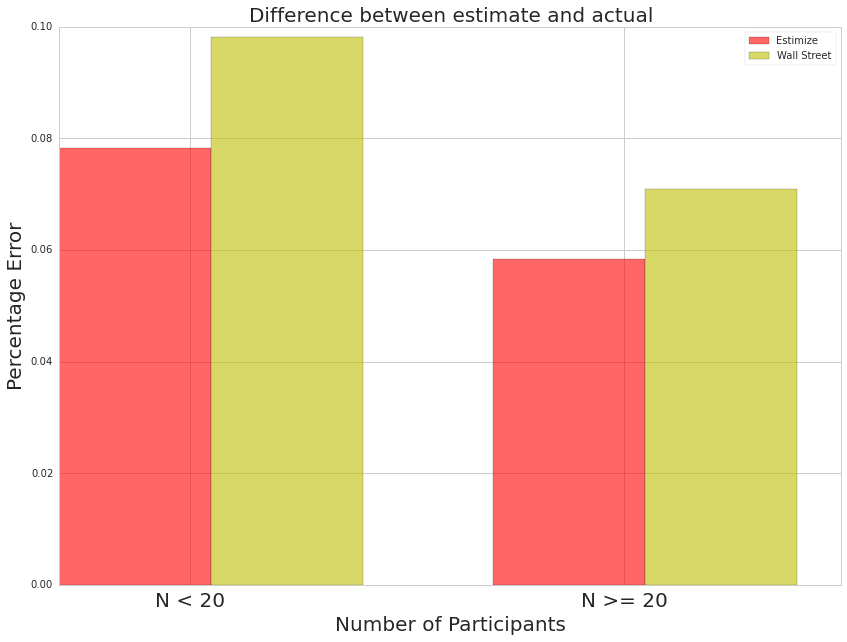There are a couple things I learned from the conclusions above: the first is that both Estimize and Wall Street's consensus numbers are less accurate when N < 20. My hypothesis is that N < 20 for companies that are relatively less known and haven't reached a critical threshold of popularity in order to enter into the retail investor's mind (e.g. AAPL has an N much greater than 20 and that's because it's popular enough for people constantly think about). And while that popularity threshold applies for Estimize (non-professional contributor) it also might mean that these same securities receive less coverage from the Street. Hence, both the Street and Estimize receive less overall estimates for these securities.

The second lesson is that, on a relative scale, Estimize's number seem to have a lower average error rate than the Street of about 1.5%. And to summarize what I've learned so far:

• N = 20 is the point at which the signal becomes significant

Now, it looks like I have a good filter to use for my data (N >= 20) before I construct an algorithm to test this event as a trading signal.

## Goals¶

• Import the backtest results from an algorithm written in the Quantopian IDE and test it's quality against a number of different risk metrics

• "The tendency for a stock's cumulative abnormal returns to drift in the direction of an earnings surprise for several weeks"

The Strategy:

• If earnings announcements are greater than estimates (Buy and Exit after 3 day)
• If earnings announcements are less than estimates (Sell and Exit after 3 day)
• Estimates are either the Wall Street Consensus numbers or the Estimize Consensus numbers
• Use only trades where the number of participants are greater than or equal to 20

The two backtests that you're about to see belong to two different strategies. The first is an algorithm that only trades on Estimize earnings surprises that are between one and eight percent. The second is an algorithm that trades on Estimize earning surprises that are between one and five percent. I compare both in order to see whether or not a bigger or smaller band makes for a better trading strategy.

In :
"""
Getting the backtest results
"""
estimize_backtest_results = get_backtest('5462498c7f087e188c09709e')
estimize_backtest_results_2 = get_backtest('546f82eb5db04a08fe00a350')
estimize_backtest_results_2.cumulative_performance.ending_portfolio_value.plot(label='1-8%')
estimize_backtest_results.cumulative_performance.ending_portfolio_value.plot(label='1-5%')
plt.title("Ending portfolio value of two different backtests")
plt.legend()

100% Time: 0:00:02|###########################################################|
100% Time: 0:00:02|###########################################################|

Out:
<matplotlib.legend.Legend at 0x7ff9eb841450>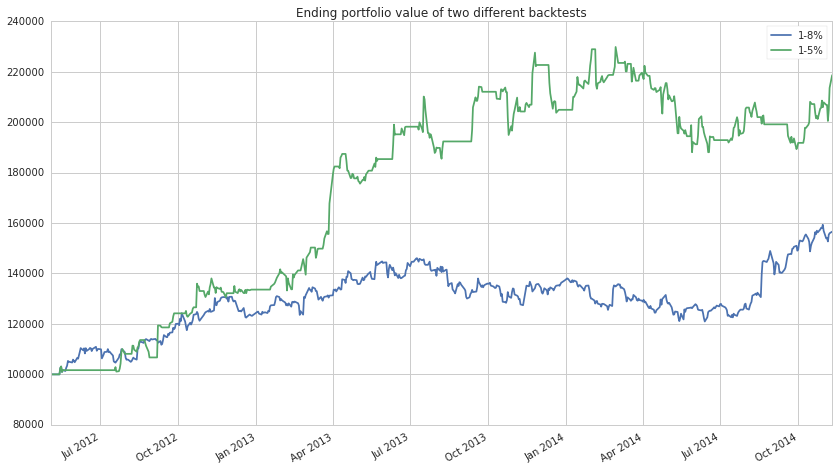In :
"""
Analyzing the strategy's Sharpe, Drawdown, and Overall Returns
"""

#: Creating the labels
drawdowns = {}
drawdowns['Estimize 1-5%'] = estimize_backtest_results.risk.max_drawdown.iloc[-1]
drawdowns['Estimize 1-8%'] = estimize_backtest_results_2.risk.max_drawdown.iloc[-1]

drawdown_labels = sorted(drawdowns.keys(), key=lambda x: drawdowns[x])
drawdown_y_pos = np.arange(len(drawdown_labels))
drawdown = [drawdowns[s]*100 for s in drawdown_labels]

avg_return = {}
avg_return['Estimize 1-5%'] = estimize_backtest_results.daily_performance.returns.mean()
avg_return['Estimize 1-8%'] = estimize_backtest_results_2.daily_performance.returns.mean()

return_labels = sorted(avg_return.keys(), key=lambda x: avg_return[x])
return_y_pos = np.arange(len(return_labels))
avg_returns = [avg_return[s]*100 for s in return_labels]

sharpe_ratios = {}
sharpe_ratios['Estimize 1-5%'] = estimize_backtest_results.risk.sharpe[-1]
sharpe_ratios['Estimize 1-8%'] = estimize_backtest_results_2.risk.sharpe[-1]

labels = sorted(sharpe_ratios.keys(), key=lambda x: sharpe_ratios[x])
y_pos = np.arange(len(labels))
sharpes = [sharpe_ratios[s] for s in labels]

#: Creating the subplots
fig = pyplot.figure()

ax.grid(b=False)
ax.barh(return_y_pos, avg_returns, align='center', alpha=0.6, color='green')
pyplot.yticks(return_y_pos, return_labels)
pyplot.xlabel("% Daily Return")
pyplot.title("Average Daily Returns")

ax.grid(b=False)
ax.barh(y_pos, sharpes, align='center', alpha=0.8)
pyplot.yticks(y_pos, labels)
pyplot.title("Sharpe Ratios")

ax.grid(b=False)
pyplot.barh(drawdown_y_pos, drawdown, align='center', alpha=0.8, color='red')
pyplot.yticks(drawdown_y_pos, drawdown_labels)
pyplot.xlabel("% Drawdown")
pyplot.title("Max Drawdown")